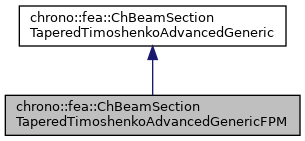## Description

Base class for all constitutive models of fully-populated matrix(FPM) sections of Tapered Timoshenko beams.

This tapered FPM section consists of two objects of ChBeamSectionTimoshenkoAdvancedGenericFPM. To be used with ChElementBeamTaperedTimoshenkoFPM.

#include <ChBeamSectionTaperedTimoshenkoFPM.h>[legend][legend]

## Public Member Functions

void SetSectionA (std::shared_ptr< ChBeamSectionTimoshenkoAdvancedGenericFPM > my_material)
Set the FPM section & material of beam element at end A.

void SetSectionB (std::shared_ptr< ChBeamSectionTimoshenkoAdvancedGenericFPM > my_material)
Set the FPM section & material of beam element at end B.

Get the FPM section & material of beam element at end A.

Get the FPM section & material of beam element at end B.

ChMatrixNM< double, 6, 6 > GetAverageFPM ()
Get the average FPM(stiffness FPM) of beam element with two ends.

ChMatrixNM< double, 6, 6 > GetAverageKlaw ()
Get the average stiffness FPM of beam element with two ends.

ChMatrixNM< double, 6, 6 > GetAverageMlaw ()
Get the average mass FPM of beam element with two ends.

ChMatrixNM< double, 6, 6 > GetKlawAtPoint (const double eta)
Get the stiffness FPM of beam element at abscissa eta.

ChMatrixNM< double, 6, 6 > GetMlawAtPoint (const double eta)
Get the mass FPM of beam element at abscissa eta.

ChMatrixNM< double, 6, 6 > GetRlawAtPoint (const double eta)
Get the damping FPM of beam element at abscissa eta.

virtual void ComputeInertiaMatrix (ChMatrixDynamic<> &M)
Compute the 12x12 sectional inertia matrix, as in {x_momentum,w_momentum}=[Mm]{xvel,wvel} The matrix is computed in the material reference (i.e. More...Public Member Functions inherited from chrono::fea::ChBeamSectionTaperedTimoshenkoAdvancedGeneric
void SetLength (double mv)
Set the length of beam element with two sections.

double GetLength () const
Get the length of beam element with two sections.

void SetLumpedMassMatrixType (bool mv)
Set the type of mass matrix: More...

bool GetLumpedMassMatrixType () const
Get the type of mass matrix： More...

void SetSectionA (std::shared_ptr< ChBeamSectionTimoshenkoAdvancedGeneric > my_material)
Set the section & material of beam element at end A.

void SetSectionB (std::shared_ptr< ChBeamSectionTimoshenkoAdvancedGeneric > my_material)
Set the section & material of beam element at end B.

Get the section & material of the element at end A.

Get the section & material of the element at end B.

virtual void ComputeInertiaDampingMatrix (ChMatrixNM< double, 12, 12 > &Ri, const ChVector<> &mW_A, const ChVector<> &mW_B)
Compute the 12x12 local inertial-damping (gyroscopic damping) matrix. More...

virtual void ComputeInertiaStiffnessMatrix (ChMatrixNM< double, 12, 12 > &Ki, const ChVector<> &mWvel_A, const ChVector<> &mWacc_A, const ChVector<> &mXacc_A, const ChVector<> &mWvel_B, const ChVector<> &mWacc_B, const ChVector<> &mXacc_B)
Compute the 12x12 local inertial-stiffness matrix. More...

virtual DampingCoefficients GetBeamRaleyghDamping () const
Get the average damping parameters of this tapered cross-section.

virtual void ComputeAverageSectionParameters ()
Compute the average section parameters: mass, inertia and rigidity, etc.

virtual std::shared_ptr< AverageSectionParametersGetAverageSectionParameters () const
Get the average sectional parameters(mass, inertia and rigidity, etc.) of this tapered cross-section.

## Protected Member Functions

virtual void ComputeAverageFPM ()
Compute the average stiffness FPM of cross-section.Protected Member Functions inherited from chrono::fea::ChBeamSectionTaperedTimoshenkoAdvancedGeneric
virtual void ComputeLumpedInertiaMatrix (ChMatrixNM< double, 12, 12 > &M)
Compute the 12x12 sectional inertia matrix in lumped format, as in {x_momentum,w_momentum}=[Mm]{xvel,wvel} The matrix is computed in the material reference (i.e. More...

virtual void ComputeSimpleConsistentInertiaMatrix (ChMatrixNM< double, 12, 12 > &M)

virtual void ComputeConsistentInertiaMatrix (ChMatrixNM< double, 12, 12 > &M)
A very generic implementation for consistent mass matrix, considering mass center offset and axis orientation. More...

## Protected Attributes

The FPM section & material of beam element at end A.

The FPM section & material of beam element at end B.

ChMatrixNM< double, 6, 6 > average_fpm
Some important average section parameters, to calculate once, enable to access them conveniently.Protected Attributes inherited from chrono::fea::ChBeamSectionTaperedTimoshenkoAdvancedGeneric
double length
The length of beam element with two sections.

The section & material of beam element at end A.

The section & material of beam element at end B.

bool use_lumped_mass_matrix
The type of mass matrix: More...

std::shared_ptr< AverageSectionParametersavg_sec_parPublic Attributes inherited from chrono::fea::ChBeamSectionTaperedTimoshenkoAdvancedGeneric
bool compute_inertia_damping_matrix = true
Flag that turns on/off the computation of the [Ri] 'gyroscopic' inertial damping matrix. More...

bool compute_inertia_stiffness_matrix = true
Flag that turns on/off the computation of the [Ki] inertial stiffness matrix. More...

bool compute_Ri_Ki_by_num_diff = false
Flag for computing the Ri and Ki matrices via numerical differentiation even if an analytical expression is provided. More...

bool compute_ave_sec_par = false
A lock to avoid computing avg_sec_par several times, initialized as false by default. More...

## ◆ ComputeInertiaMatrix()

 void chrono::fea::ChBeamSectionTaperedTimoshenkoAdvancedGenericFPM::ComputeInertiaMatrix ( ChMatrixDynamic<> & M )
virtual

Compute the 12x12 sectional inertia matrix, as in {x_momentum,w_momentum}=[Mm]{xvel,wvel} The matrix is computed in the material reference (i.e.

it is the sectional mass matrix)

Parameters
 M 12x12 sectional mass matrix values here